# How to Limit the Number of Decimal Places in Excel

This post will guide you how to limit the number of decimal places in Excel. How do I round a number to the specific decimal places with a formula in Excel. How to Limit Number of decimal places with Format Cell Feature in Excel.

## Limit Number of Decimal Places with in Formula

Assuming that you have a list of data in range B1:B5, in which contain number values with 3-4 decimal places. And you want to limit the number of decimal places for all numeric values to 2. How to do it. You can use the ROUND function to create a formula to limit the number of decimal places for each numbers. So you can use the following formula:

`=ROUND(B1,2)`

Type this formula into the Cell C1, and press Enter key on your keyboard, and then copy this formula from Cell C1 to the range C2:C5 to apply this formula.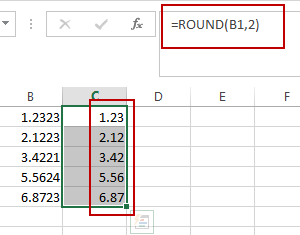You should see that all numbers have been limited to two decimal places.

## Limit Number of Decimal Places with Format Cell

You can also use the Format Cell feature to achieve the same result of limiting the number of decimal places for the selected range that contain numbers. Just do the following steps:

#1 select the range of cells that contain numbers with four decimal places.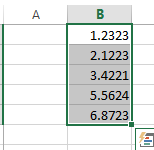#2 right click on it, and select the Format Cells from the popup menu list. And the Format Cells dialog will open.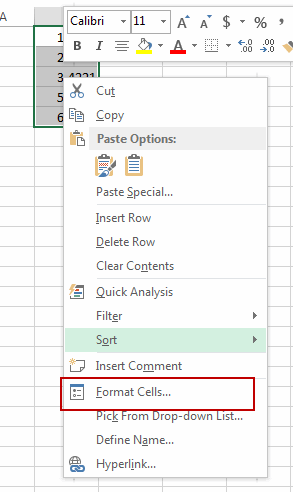#3 click the Number tab in the Format Cells dialog box, and click the Number option in the Category list box, and then type number 2 in the Decimal places box. Click Ok button.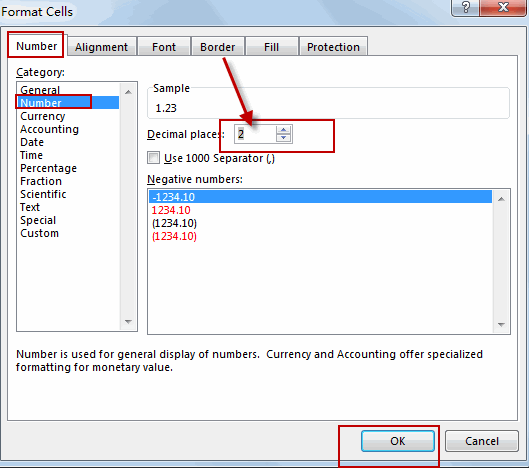#4 you should notice that all numbers in the selected range have been changed to two decimal places.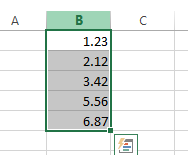### Related Functions

• Excel Round function
The Excel INT function rounds a number to a specified number of digits. You can use the ROUND function to round to the left or right of the decimal point in Excel.The syntax of the ROUND function is as below:=ROUND (number, num_digits)…